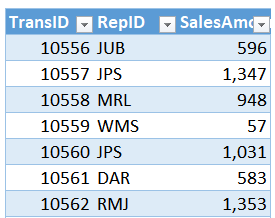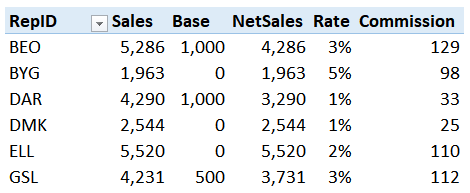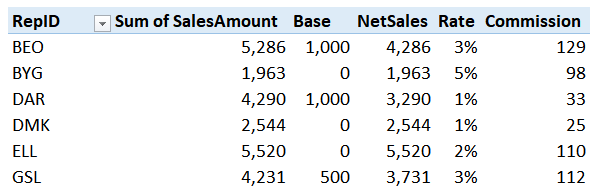# Impossible PivotTables 1 – Calculated FieldsThis is the first post in a series called Impossible PivotTables. The purpose of this series is to explore Power Pivot. I thought a fun way to do that would be to demonstrate how using the data model enables us to build PivotTables that are either impossible with traditional PivotTables or that require workarounds. I hope it provides an enjoyable way to examine Power Pivot 🙂

## Impossible PivotTables?

Historically, when I tried to build a traditional PivotTable (PT) that wasn’t supported by Excel, I’d have to figure out some type of workaround. These workarounds weren’t always pretty, but, they helped me get the numbers I needed. I’d use workarounds like adding helper columns to the data table, copy/pasting multiple data tables into a single data source table, clicking-and-dragging to manually sort the labels, or creating formulas outside the PT on the worksheet. All of these worked, sort-of, but, they didn’t feel very elegant. They didn’t feel very reliable either … they felt fragile, like they could easily break in future periods when I had to update the report. Then, everything changed when I learned about Power Pivot (PP).

Power Pivot essentially allows us to combine the mathematical ability of formula-based reports with the PivotTable feature. It allows us to build PT reports that don’t require the workarounds mentioned above. The result is a clean, reliable report that is easy to update and maintain over time. And, honestly, they just feel better.

So, enough background jibber-jabber, let’s go build our first impossible PivotTable.

## Objective

Traditional PivotTables are great at summarizing and aggregating values that are stored within a data source table. When you need your report to compute values that aren’t included within the data source, you can create Calculated Fields. However, this feature is not very robust and has limitations. For example, a calculated field can operate on values within the report, but not on values outside of the report in another range or table. So, when we encounter this limitation, we try to work around it. For example, we may add a helper column to the data table or decide to perform the calculations outside of the PT. But, these workarounds have issues. For example, adding a helper column in the data table may not provide the desired math in a given report. That is, the math may need to operate on aggregated subtotals or totals rather than on each row. And when we create formulas outside of the PT, they aren’t refreshed along with the PT … meaning we need to babysit them to be sure they are filled down for new rows.

To illustrate this issue, I’ll provide an example report that computes commission based on sales data. Let’s say we have a bunch of sales transactions, as shown below.Then, we have each of the rep’s commission rates and base values in another table, as shown below.The report we’d like to create will add up the sales transactions, subtract the base sales amount, and then multiply the resulting net sales amount by the corresponding commission rate. We are after something like this:Before we even start building the report with a traditional PT, we encounter a problem. A traditional PT supports a single source data table, but our data comes in two tables. But, let’s set that fact aside for the moment and focus on what we can do. We can easily use a traditional PT to summarize the sales by rep, so we start with that. We create the PT and insert the RepID and Sales fields.

Next, we try to create a Calculated Field to compute the commission values. The formula would basically use VLOOKUP to retrieve the commission rate and base amount for each rep. We open the Calculated Field dialog and when we enter a formula that tries to reference values outside of the PT, such as the commission rates table, we receive the following error message:So, we quickly conclude this is an impossible PivotTable and try to come up with a clever workaround. For example, we try using a helper column in the data table to retrieve the commission rates. And that works, but when we go to compute the commission amounts, we realize that we need to aggregate the sales values and subtract the base before applying the rate. So, we hit a dead-end with that and try something else.

We proceed to compute commission outside of the PT in normal Excel cells. When we do this, the final report isn’t even a PT … it is a formula-based report that references an intermediate PT for the aggregated sales values. And while it provides the numbers we need for this month, what about next month? When we think ahead, we realize that this approach is fragile and may break next period when we update the report. When formulas are written outside the PT, they won’t be included when the PT is refreshed. So, hopefully we’ll remember to fill the formulas down manually to include any new reps. And, as you may imagine, this is where Power Pivot comes in to help us out.

This PivotTable is possible when we use Power Pivot instead of a traditional PivotTable… and no workarounds are needed 🙂

## Overview

We’ll build this PivotTable using the following steps:

• Load the tables into the data model
• Build the basic PivotTable
• Write the measures

Let’s get to it.

Note: The steps below are presented with Excel for Windows 2016. If you are using a different version of Excel, please note that the features presented may not be available or you may need to download and install the Power Pivot Add-in.

## Load the tables into the data model

First up, we need to load the tables into the data model and relate them. To do this, we select any cell in our commission rates table and click the Power Pivot > Add to Data Model command.

We do it again for the table that stores the sales transactions. Select any cell in the data table and click the Power Pivot > Add to Data Model command.

My favorite way to relate these two tables is by using diagram view, so, inside the Power Pivot window, we click Home > Diagram View. We can see the two tables, as shown below.Next, we need to tell Excel how these tables are related to each other, that is, which column is shared between them. In our case, it is the RepID column. So, we click-and-drag the RepID from one table to the other. Excel adds the relationship line, as shown below.With this complete, it is time to build our basic PT.

## Build the basic PivotTable

To get our PivotTable started, we use Excel’s Insert > PivotTable command. In the resulting dialog, we want to Use this workbook’s Data Model, as shown below.Next, we insert the CommissionRates[RepID] field into the Rows area, and the Transactions[SalesAmount] and CommissionRates[Base] fields into the Values area. The basic report is shown below.With our basic PT looking good, it is time to do the remaining calculations by writing a couple measures.

## Write the measures

First, we need to subtract the base sales from the sum of sales to determine the commissionable net sales amount. To do this, we use the Power Pivot > Measures > New Measure command. In the resulting dialog, we enter the desired measure name, NetSales, and the corresponding formula as shown below.Then, we repeat the steps to create our next measure, Commission, which multiplies the NetSales measure by the commission rate, as shown below.We can toss the NetSales measure, the Rate field, and the Commission measure into the values area of the PivotTable, and the updated report is shown below.And look … no workarounds in sight. It is like Power Pivot made an impossible PivotTable possible 🙂

If you’d like to investigate the details, please check out the sample file below. And if you have any other fun Power Pivot tips, please share by posting a comment below.

## Sample File

Get a quick email notice when a new Excel article is available

• This field is for validation purposes and should be left unchanged.1.Alan Maxwell

Hi Jeff, thank you covering Power Pivots – With new functionality being included in each new version of Excel, it is not easy keeping up.

The ‘Grand Total’ row shows:
Sales = 71,475
Base = 14,500
Net Sales = 56,975
Rate = 39% (*** this reporting the sum of the individual [Rates] looks odd)
Commission = 22,220
It looks like the [Commission] grand total amount is calculated as grand total [Net Sales] x grand total [Rate], when I think it should be the sum of the individual Sales Rep commission amounts and total 1,471.68.
This gives a ‘Grand Total’ rate of 1471.68 / 56,975 or 2.58%, not 39%.

Can you confirm what the total commission should be?

Note: The approach I used was array formulas. They have their own benefits and issues when compared to Pivot Tables and Power Pivots, but it is a useful item in my toolbox,

1.Jeff Lenning Post author

Alan … great catch, thank you. I’ve updated the sample file and renamed it Commission2.xlsx which addresses the grand total issue you spotted. I used a couple of extra DAX functions to get the grand total displays as desired.
Thanks
Jeff

2.Jean-Sébastien

I saw the same problem as Alan.

I did a pivot table to practice but mine gives the 39% and 22,220 instead of an empty cell and 1,472 as yours.<

I can't figure how you got there.

1.Jeff Lenning Post author

For the commission measure, I used the SUMX function to iterate through the RepID values adding up the results, and for the rate measure, I hid the grand total by using the BLANK function … these updated measures are provided in the Commission2 sample workbook in case you’d like download and check them out…thanks!
Thanks
Jeff

3.Joan Hauff

My Excel 2013 (Microsoft Office Professional Plus) does not have the Use this workbook’s Data Model option under Insert > PivotTable.
Is there a workaround?

1.Jeff Lenning Post author

In that case, you may want to insert the PivotTable using the Power Pivot window’s command (rather than Excel’s).
Thanks
Jeff

4.Moshe Slonim

​​Hi Jeff,
First, thank you for the useful information you send. It’s very helpful.
In a pivot table, I have column ​D with annual sales results.
I wish to calculate the annual change percentage.
In a regular Excel file it would look like this:
=(D20-D19)/D19
I cannot figure out how to use DAX formula to divide 2 cells one above
the other.
Thanks

5.Mario

Hi all, I check the new workbook Commsions2.xls and follow along the post. I came with the same issues for the totals (rates and commissions). I tried to figure out the total for the commissions from the file and found the calculating field a little confusing and did some research. The research was based on the following question How do I sum the value of two or more Measures together in Power Pivot? I found which I believe is an easier way to get both individual commissions and total for commission column in the Pivot Table.

Solution: To add two or more measures since there is no DAX SumProduct formula and SUMX needs a table column to work Jeff use a measures for commissions and use the followings DAX Formula:

1) Commission:=[NetSales]*[C_rate] – Measures that calculate the commissions for each sales RepID not showing in the pivot table.

2) Commission_total:=SUMX(VALUES(CommissionRates[RepID]),[Commission]) – DAX function that agreegate the Commissions from 1) above.

Here is which I believe may be a better way to get the same result that is getting together both formulas into one:

1) Commissions:=SUMX(DISTINC(CommissionRates[RepID]), [NetSales]* [Sum of Rate]) – this way the SUMX DAX function calculate every instance of commission calculation for each RepID and adds up all of them. Notice the only one calculated field is needed to show in the pivot table both the individual RepID commissions and the total commissions. The SUMX includes the measure performed in Commission from 1) above and is included inside the formula.

For the grand total for commission rate I simply create the following calculated field which calculate the total average rate that should be 2.58302764370338% (calculated using Goal Seek) using two SUMX DAX formulas:

1) Commissions Rate:=DIVIDE(SUMX(DISTINCT(tblCommissionRates[RepID]),[Commissions])/SUMX(DISTINCT(tblCommissionRates[RepID]),[NetSales])) – it shows 2.58%.

a) 1st SUMX formula gets each value for every measures record incuded in the formula [Commissions] each record was the commission calculated for each RepID and calculate the aggregate of all commissions (the key part of the formula);

b) 2nd SUMX formula gets each value for every measures record included in the formula [NetSales] each record represents Sales minus the Base the amount to be used to calculate the commissions and calculate the aggregate of all Net Sales (the key part of the formula;

c) 3rd the DIVIDE function perform the recalculation of the Rates that should be presented for each RepID and the exact total rate is calculated since SUMX function agreegate both valued needed as this: [Commissions]/[NetSales]= Commissions Rates.

To understand how it is perform the key part is that each SUMX function performs two operations:

1. Presents each record individually for the calculated expression or individual values and;

2. Aggregates the total of every record presented and calculated or individual values from “Expression” part of the SUMX function.

Accordingly, the “SUMX” Function nested with “DIVIDE” function (only perform division, numeratot/denominator) calculates backwards the rates to be presented for each individual RepID and because aggregates at the same time it ends up calculating the total rate at the end that is included in the pivot table.

Thank you Jeff ! Your post make me to practice Power Pivot and learn more about the formulas in Power Pivot and how they works.

1.Jeff Lenning Post author

Wonderful comment … thanks!

6.William

Hi Jeff,
It’s 2019 now, and I’m not sure if you are still around.
What if our boss wants to see those sales number by months, and is there a way to combine those month like 2018 YTD and 2019 YTD?
I know pivot table’s calculated items can solve it, but it takes a lot of time to run.
I’m not sure if power pivot has this function.
1.Jeff Lenning Post author